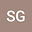Inversion of Two-dimensional Electrical Resistivity data using Convolutional Neural Networks (U-Net
•••• +2Deepak Devpal
Divakar Vashisth
Ved Prakash Maurya
Subash Chandra

## Abstract

The interpretation of ERT data requires inversion, which is challenging due to its nonlinear and ill­posed nature. We aim to solve the inverse problem using a machine learning technique that approximates the complex non-linearity using the data provided without specifying any geophysical or mathematical equations. Speci¦cally, we make use of CNN with U-Net architecture to estimate the true subsurface resistivity model from observed apparent resistivity data (pseudosection). U-Net is selected because it works well when both input and output data are in the form of images and in the present work, input (pseudosection) and output (subsurface resistivity model) have spatial correspondence and existing local patterns.
For ERT inversion, U-Net learns by minimizing pixel mean squared error between the true and predicted subsurface resistivity images. The ERT dataset was generated by simulating a dipole­dipole survey over a 400m x 100m earth containing two anomalous blocks with varying resistivity, size, and position in a variable homogeneous background resistivity. We used a ¦nite element approach with a triangular mesh grid for forward modelling (using pyGIMLi) and added Gaussian noise to the calculated pseudosection so that simulated data resembles the ¦eld data.
The U-Net model was trained on 4000 samples (20% of training samples used for validation) and tested on 1000 samples. The architecture consisted of an encoder and decoder subnetwork connected by a bridge section. The encoder depth was 7, and each block in the encoder subnetwork consisted of two sets of convolutional and ReLU layers, followed by a max pooling layer. The decoder subnetwork consisted of a transposed convolution layer for upsampling, followed by two sets of convolutional and ReLU layers. The weights of all the convolutional layers were initialized using the He Normal initializer. We used Adam optimizer with a learning rate of 0.0001. The U-Net model was allowed to train for 100 epochs with a batch size of 128. The trained U-Net model gave good predictions of the anomalous blocks, and background resistivity with a root mean square mis¦t of 2.86% on test data and 2% on training data. The results con¦rm the e¨cacy and effectiveness of the proposed U-Net architecture in predicting the subsurface resistivity variation from the DC resistivity data.
04 Jan 2023Submitted to AGU Fall Meeting 2022
09 Jan 2023Published in AGU Fall Meeting 2022